• Introduction
• What is in this manual
• What is Caspoc
• User interface
• Introduction
• Starting
• Simulation
• Editing
• Viewing and printing
• Getting Started
• Basic editing
• Simulation in the time domain
• Basic User Interface Topics
• Editing
• Simulation
• Viewing
• Library
• Reports
• Project management
• Circuit and Block Diagram Components
• Introduction
• Cscript and user defined functions
• Component parameters
• Modeling Topics
• Introduction
• Power Electronics
• Semiconductors
• Electrical Machines
• Electrical drives
• Power Systems
• Mechanical Systems
• Thermal Systems
• Magnetic Circuits
• Green Energy
• Coupling to FEM
• Experimenter
• Analog hardware description language
• Embedded C code Export
• Coupling to Spice
• Small Signal Analysis
• Matlab coupling
• Tips and tricks
• Appendices

## .Model Database Parameters.

When you create your own models you also specify parameters for these models. If you would like to use the model with various parameter sets, the .Model database is a very simple solution. You can provide different parameters sets by assigning only the .Model database name.

Parameters are usually defined in "CON" blocks, but it is more convenient to store them in a database. In this section we will discuss how to use the .Model database to create user specific models.

Suppose we would need 2 parameters to create the offset:

  y=a*(input)+b 

Open the commands editor by selecting "Tools/Commands Editor"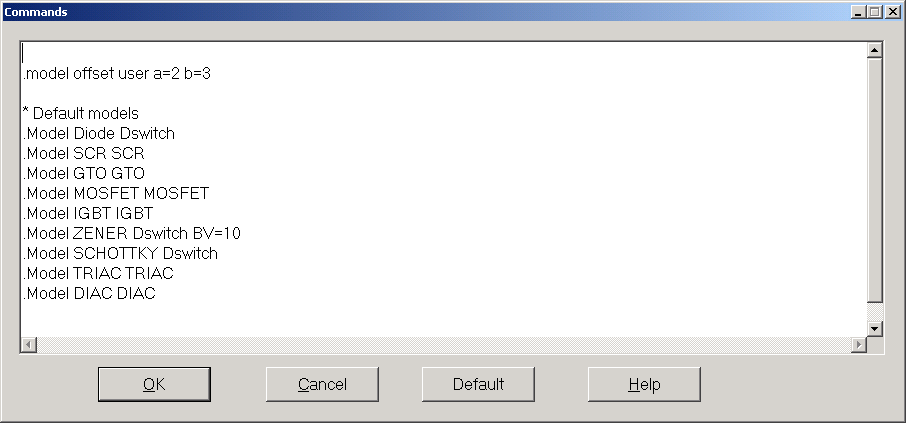.model offset user a=2 b=3 

.model offset user a=2 b=3

The model "offset" must be of the type "user" if we want to have access to a and b.

Create a block diagram as shown below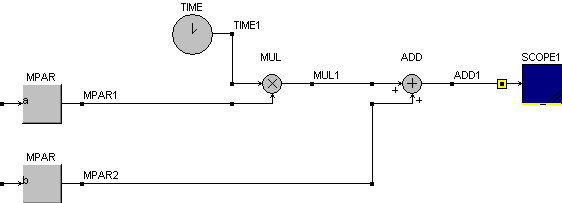In the block "MPAR1" define "a" in the edit field "Text1" and "offset" in the "text2" edit field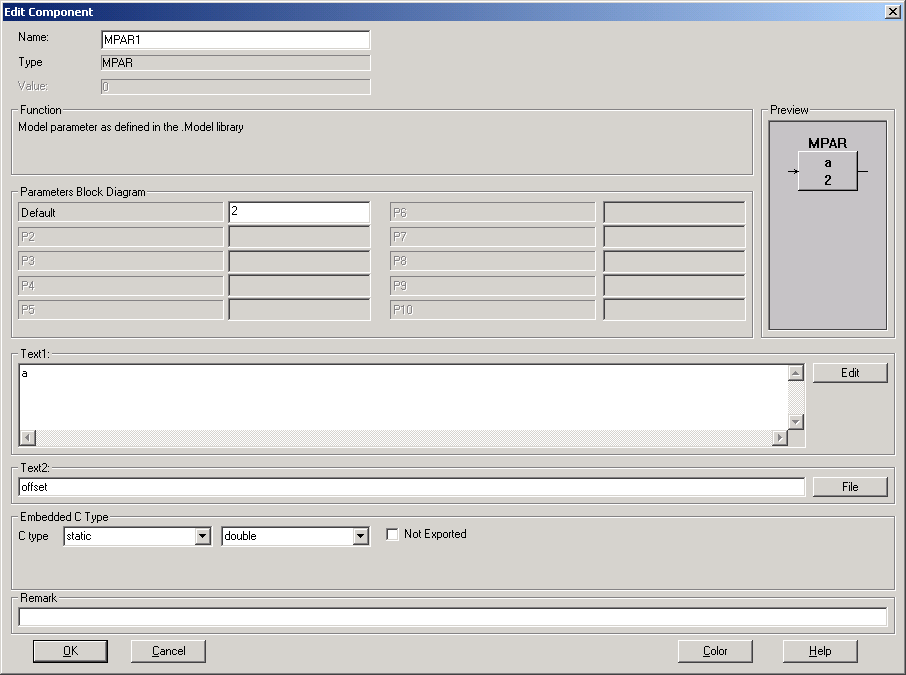A default value can be defined at p1 in case the parameter a in the offset model is not found. In the block MPAR2 define "b" in the edit field "Text1" and "offset" in the "text2" edit field. Also a default value can be specified at p1.

The inputs of both MPAR block can be left undefined. Running the simulation with Tscreen=10s and a stepsize dt=10ms gives the results as shown below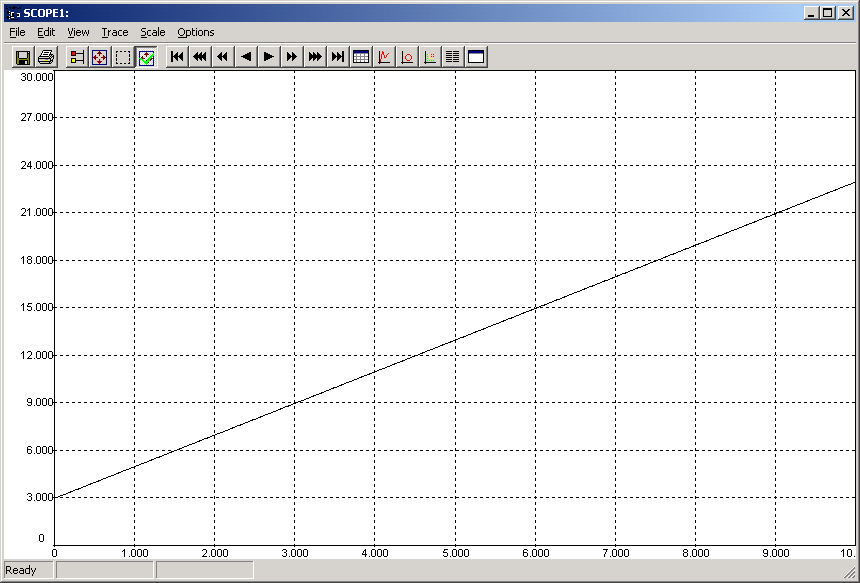Suppose we want to create another offset, we could change the values in the .model line. We can also add more offset models and select them by modelname. Add the following lines in the command editor:

  .model offset1 user a=1 b=3 .model offset2 user a=2 b=3 .model offset3 user a=3 b=4 

Adding a "MNAME" block to the block diagram as shown below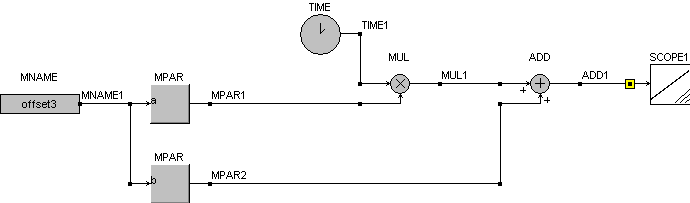Starting the simulation with Tscreen=10 and dt=10ms gives the offset specified by model offset3. By changing the model name in the "text1" edit field in the block"MNAME", changes the user model.